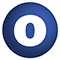# RDDHistogramEqualization

### Related Doc: package equalization

#### object RDDHistogramEqualization

Linear Supertypes
AnyRef, Any
Ordering
1. Alphabetic
2. By Inheritance
Inherited
1. RDDHistogramEqualization
2. AnyRef
3. Any
1. Hide All
2. Show All
Visibility
1. Public
2. All

### Value Members

1. #### final def !=(arg0: Any): Boolean

Definition Classes
AnyRef → Any
2. #### final def ##(): Int

Definition Classes
AnyRef → Any
3. #### final def ==(arg0: Any): Boolean

Definition Classes
AnyRef → Any
4. #### final def asInstanceOf[T0]: T0

Definition Classes
Any
5. #### def clone(): AnyRef

Attributes
protected[java.lang]
Definition Classes
AnyRef
Annotations
@throws( ... )
6. #### final def eq(arg0: AnyRef): Boolean

Definition Classes
AnyRef
7. #### def equals(arg0: Any): Boolean

Definition Classes
AnyRef → Any
8. #### def finalize(): Unit

Attributes
protected[java.lang]
Definition Classes
AnyRef
Annotations
@throws( classOf[java.lang.Throwable] )
9. #### final def getClass(): Class[_]

Definition Classes
AnyRef → Any
10. #### def hashCode(): Int

Definition Classes
AnyRef → Any
11. #### final def isInstanceOf[T0]: Boolean

Definition Classes
Any
12. #### def multiband[K, V, M, T <: AnyVal](rdd: RDD[(K, V)] with Metadata[M], histograms: Array[Histogram[T]])(implicit arg0: (V) ⇒ MultibandTile, arg1: ClassTag[V]): RDD[(K, MultibandTile)] with Metadata[M]

Given an RDD of MultibandTile objects and a sequence of Histogram objects (on per band) derived from all of the tiles, return another RDD of multiband tiles where the respective bands of the respective tiles have had their histograms equalized.

Given an RDD of MultibandTile objects and a sequence of Histogram objects (on per band) derived from all of the tiles, return another RDD of multiband tiles where the respective bands of the respective tiles have had their histograms equalized.

rdd

An RDD of tile objects

histograms

A histogram derived from the whole RDD of tiles

13. #### def multiband[K, V, M](rdd: RDD[(K, V)] with Metadata[M])(implicit arg0: (V) ⇒ MultibandTile, arg1: ClassTag[V]): RDD[(K, MultibandTile)] with Metadata[M]

Given an RDD of MultibandTile objects, return another RDD of multiband tiles where the respective bands of the respective tiles have been equalized according to a joint histogram of the bands of the input RDD.

Given an RDD of MultibandTile objects, return another RDD of multiband tiles where the respective bands of the respective tiles have been equalized according to a joint histogram of the bands of the input RDD.

rdd

An RDD of multiband tile objects

14. #### final def ne(arg0: AnyRef): Boolean

Definition Classes
AnyRef
15. #### final def notify(): Unit

Definition Classes
AnyRef
16. #### final def notifyAll(): Unit

Definition Classes
AnyRef
17. #### def singleband[K, V, M, T <: AnyVal](rdd: RDD[(K, V)] with Metadata[M], histogram: Histogram[T])(implicit arg0: (V) ⇒ Tile, arg1: ClassTag[V]): RDD[(K, Tile)] with Metadata[M]

Given an RDD of Tile objects and a Histogram which summarizes all of the tiles, return another RDD of tiles where the respective tiles have had their histograms equalized.

Given an RDD of Tile objects and a Histogram which summarizes all of the tiles, return another RDD of tiles where the respective tiles have had their histograms equalized.

rdd

An RDD of tile objects

histogram

A histogram derived from the whole RDD of tiles

18. #### def singleband[K, V, M](rdd: RDD[(K, V)] with Metadata[M])(implicit arg0: (V) ⇒ Tile, arg1: ClassTag[V]): RDD[(K, Tile)] with Metadata[M]

Given an RDD of Tile objects, return another RDD of tiles where the respective tiles have had their histograms equalized the joint histogram of all of the tiles.

Given an RDD of Tile objects, return another RDD of tiles where the respective tiles have had their histograms equalized the joint histogram of all of the tiles.

rdd

An RDD of tile objects

19. #### final def synchronized[T0](arg0: ⇒ T0): T0

Definition Classes
AnyRef
20. #### def toString(): String

Definition Classes
AnyRef → Any
21. #### final def wait(): Unit

Definition Classes
AnyRef
Annotations
@throws( ... )
22. #### final def wait(arg0: Long, arg1: Int): Unit

Definition Classes
AnyRef
Annotations
@throws( ... )
23. #### final def wait(arg0: Long): Unit

Definition Classes
AnyRef
Annotations
@throws( ... )